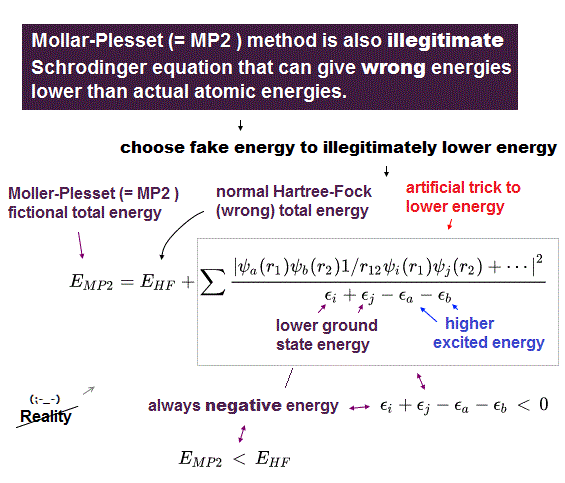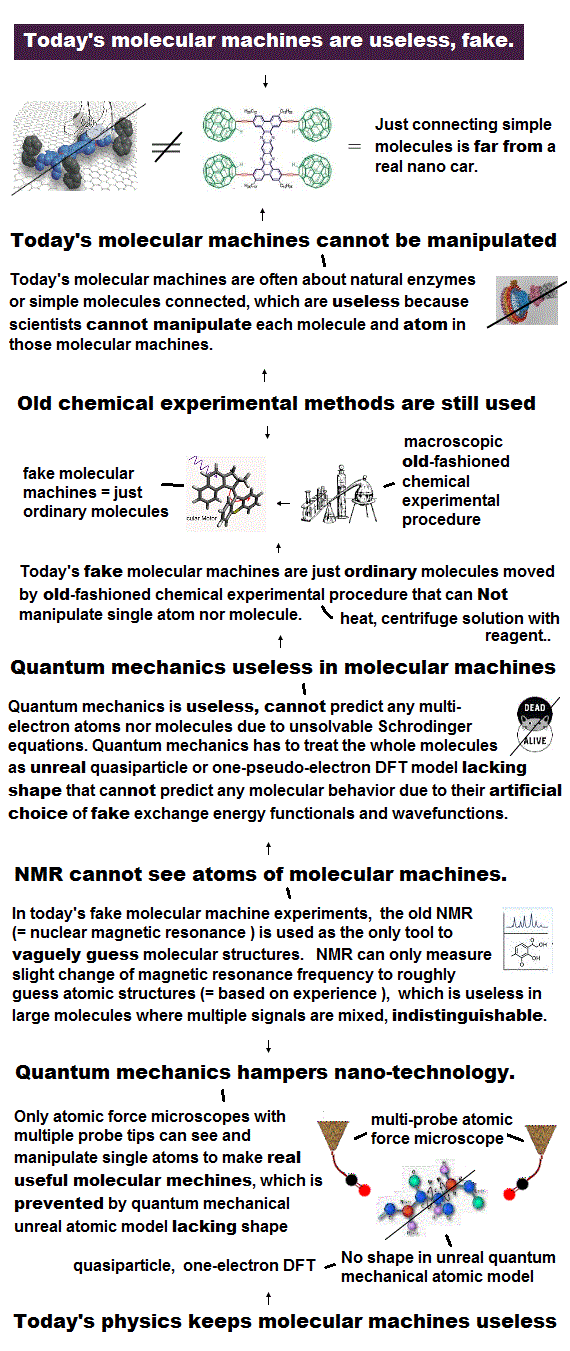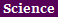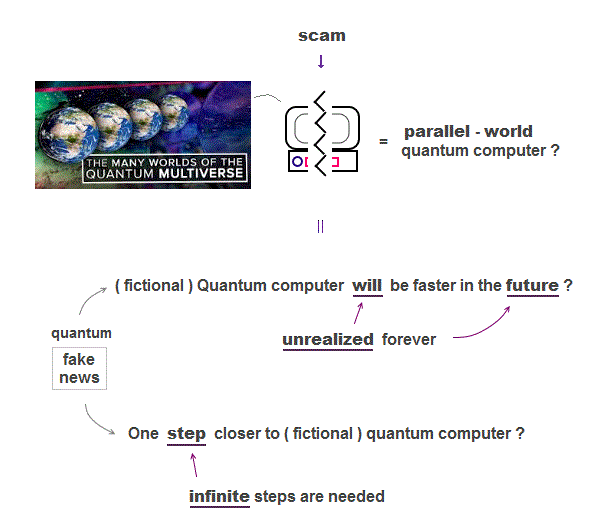﻿ Spin Pauli principle is nonphysical exchange energy.

# Pauli spin is false.

Summary

### [ Pauli exclusion principle contradicts quantum mechanics. ]

(Fig.1)  ↓ If Schrödinger equation is solvable, Pauli principle disappears.Pauli exclusion principle is uncanny like spin.

Pauli principle says two electron with the same spin cannot occupy the same orbital due to Pauli exclusion repulsion, which is a measurable real force.

But quantum mechanics never admits Pauli principle to be a real force.  Still No physical meaning of Pauli principle ( this p.6, this p.3 )

Unless we can accept Pauli principle as "real force", we cannot calculate the total force applied to each particle to predict chemical realction, because the total force must be the sum of Pauli repulsion and other Coulomb force.

This abnormal state has continued for 100 years since quantum mechanics was born !  This unknown Pauli principle is the main factor which prevents our science from advancing.

In fact, Pauli principle contradicts the original definition of quantum mechanics.  This is the reason why physicists still cannot show physical meaning of Pauli principle, except nonphysical math.

Only in one-electron hydrogen atom, Schrödinger equation of quantum mechanics has the exact solution which always gives the constant total energy E wherever an electron is.

If even two-electron atoms have exact solution (= solvable Schrödinger equation ), Pauli principle is meaningless and invalidated.

Suppose this "exact" solution of two-electron atom is " φ1ψ2 "  ( or φ2ψ1  The numbers 1, 2 are labels of electrons 1, 2.  Just exchanging the labels 1 and 2 gives the same wavefunction of the same energy E ).

Quantum mechanics claims Pauli exclusion principle is expressed as "antisymemtric wavefunction" where exchanging two electrons' labels 1 and 2 changes the sign of the total wavefunction.

This Pauli principle is just an old, nonphysical, artificial rule with No physical meaning.

The energy difference between symmetric (= + in the upper figure ) and antisymmetric (= - ) wavefunctions means Pauli exclusion energy.

But if the two-electron atom has the exact solution E, the energy difference between symmetric and antisymmetric disappears and invalidates Pauli exclusion principle.

So if Schrödinger equation is solvable, this exact solution cannot express Pauli principle.  If No exact solution is found, Schrödinger equation is false.  = self-contradiction !

Only when Schrödinger equation has No exact solution ( which means Schrödinger equation is false ), Pauli principle is effective.  This is self-contraditory and shows quantum mechanics is wrong.

## Pauli principle is nonphysical.

### [ No exact solution → integral → Pauli "fake" energy. ]

(Fig.2)  ↓ Only "unsolvable" Schrödinger equation gives Pauli energy ?Schrödinger equation of multi-electron atoms is unsolvable with No exact solution.

Physicists just choose approximate fake solution with freely-adjustable parameters, and integrate those fake solution instead of solving it ( because they cannot solve it ), which gives fake total energies E±.

Only in this unsolvable Schrödinger equation, Pauli principle appears.

Because symmetric (= E+ ) and antisymmetric (= E- ) wavefunctons give different total energies by integral (= only when Schrödinger equation is unsolvable ), that energy difference means Pauli principle ( this p.14 ).

Pauli exclusion principle is valid only when Schrödinger equation is unsolvable with No exact solution.

"Unsolvable" means No exact solution, No constant total energy = No conserved energy, which proves quantum mechanics is wrong.

The overlap integral ( of two different wavefunctions ) which gives Pauli exchange energy is not an usual electron's probability density (= integral of two same wavefunctions ), so no physical meaning (= Pauli principle cannot be expressed using real things ) !

## Quantum technology fake news.

### [ Imaginary target = "future quantum computer" ? ]

(Fig.3)  ↓ The media uses misleading phrasesQuantum mechanics is useless, so it needs to create "imaginary target = quantum computer" to deceive taxpayers and governments.

No progress in research on fictional quantum computer, which is said to be faster (← No proof ! ) using parallel-world computing.

If you carefully read each quantum technology news, you will notice they make No progress, just using misleading phrases to get your hopes up.

"A step towards", "aims to", "could lead to", "could revolutionize", "new path ?"  All these vague phrases say only about "imaginary future", Not now.

The first paragraph of this news just says "atomic Schrödinger cat states is regarded as an important step in the development of quantum computers which could outperform .. ? "  ← still useless, nothing is realized now.

A unreal dead and alive Schrödinger cat (= an atom can be in two different states at once ? ) lives only in fantasy parallel worlds which is unobservable, so No proof.  Meaningless research.

The first paragraph of this news is also about imaginary "future", saying "Quantum computing and quantum cryptography are expected to give much higher capabilities .. ?"  ← still useless.  Don't be deceived by science fake news.

Useless quantum mechanics cannot give real deeper mechanism, instead, it allows unrealistic "negative resistance ?"  =Just using eye-catching title "counterintuitive physics found .. ", this research is useless, realized nothing.2019/8/16 updated. Feel free to link to this site.